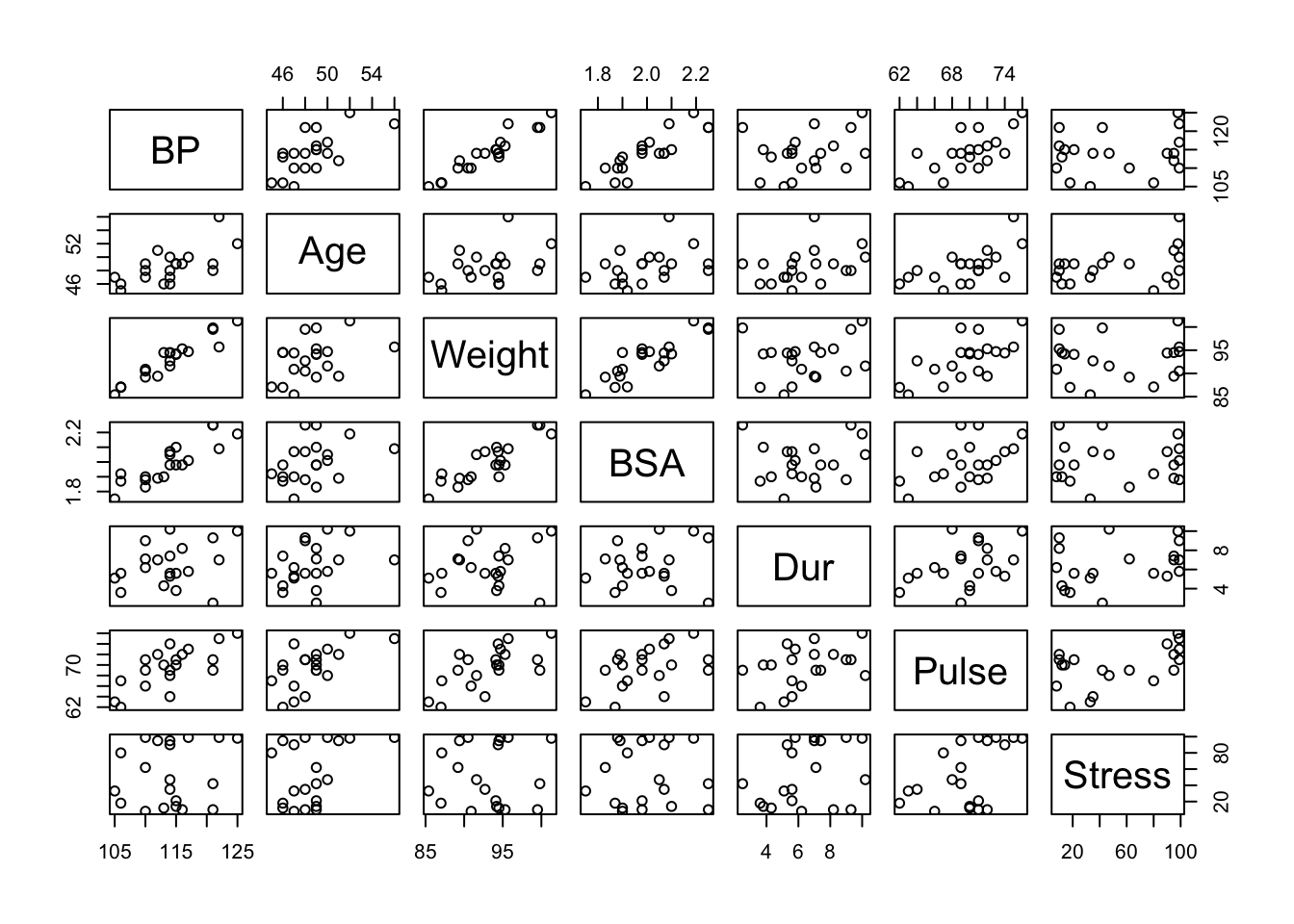``````require(mosaic)
require(car)``````

Researchers observed the following data on 20 individuals with high blood pressure:

• blood pressure (`BP`, in mm Hg)
• age (`Age`, in years)
• weight (`Weight`, in kg)
• body surface area (`BSA`, in `m^2`)
• duration of hypertension (`Dur`, in years)
• basal pulse (`Pulse`, in beats per minute)
• stress index (`Stress`)

Our goal is to build a model for blood pressure as a function of (some subset) of the other variables. In this case all of our variables are quantitative, so we can get a quick look at their relationships using the a pairs plot.

``````BP <- read.csv("http://www.math.smith.edu/~bbaumer/mth247/labs/bloodpress.csv")
pairs(BP)````````````# Better than the standard pairs plot is the generalized pairs plot.
#install.packages("gpairs")
#gpairs(BP)``````
• What do you see in these scatterplots? Which of the variables are most highly correlated with `BP`?

Hint: use `cor` to calculate the correlation coefficient matrix.

#### A First Model

`Weight` seems to be highly correlated with `BP`, so as a first step, we should understand how well a simple linear model for blood pressure as a function of weight works. Keep in mind that it accords with our intuition that there would be a strong link between a person’s weight and their blood pressure.

``xyplot(BP ~ Weight, data=BP, pch=19, alpha=0.5, cex=1.5, type=c("p", "r"))``